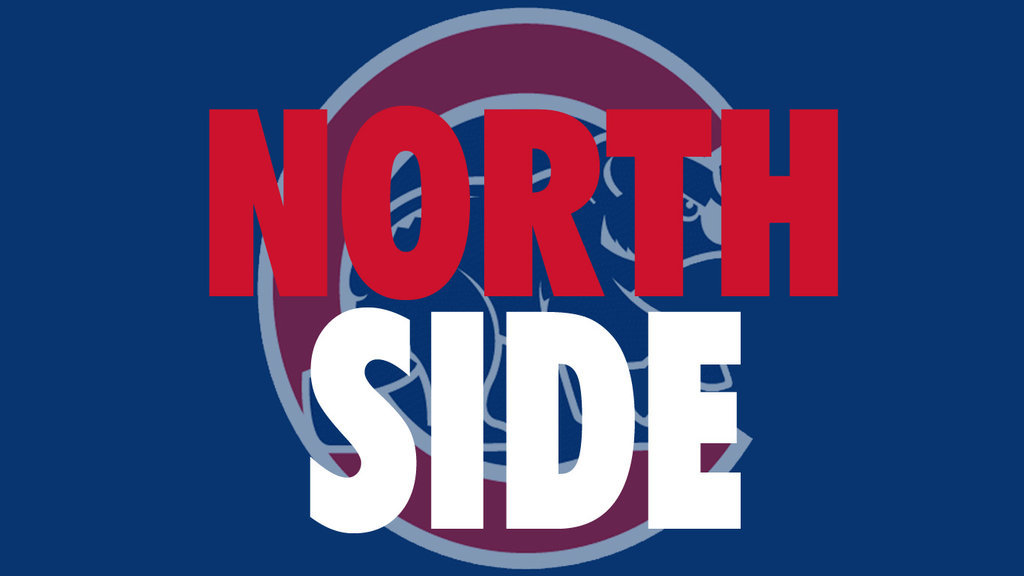Cubs vs Dodgers

Event Packets Were Distributed to Students on March 27

Cubby Walk is our annual walk-a-thon from Northside to Wrigley Field. Students raise money for lab equipment, supplies, books, colloquium resources, athletic equipment, and much more. It's an opportunity for our students to have a lot of fun while helping their school.

ONLINE DONATIONS are encouraged. Go to the Cubby Walk web page. Spread the word! Click on the link, copy the URL, and email it to your friends and family.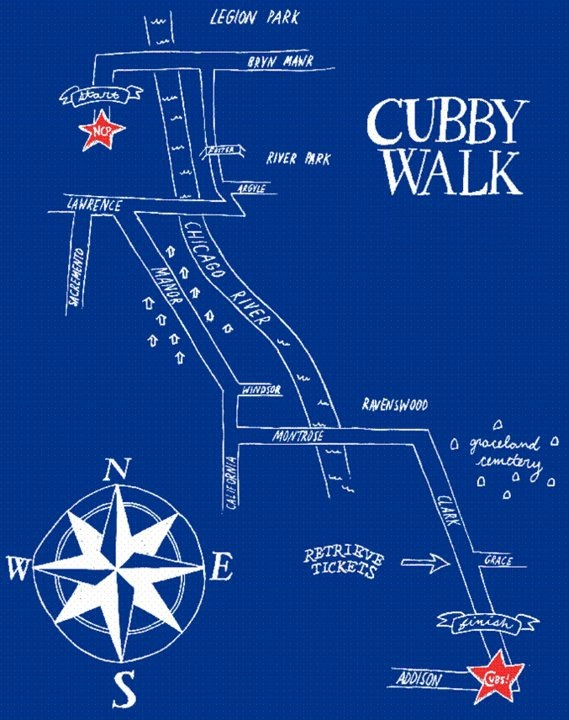A minimum donation of \$40 or more will get your student an event t-shirt (winning design from school design contest), game ticket, future off-campus lunch, and a chance to win some great raffle prizes. Pay online and receive an exclusive NCP silicone phone wallet (while supplies last)! Every student that turns in the packet envelope with a completed permission form, donation form, and payment (if not made online) by April 12, will be invited to the Ice Cream Party on May 25!Top student fundraisers for each grade level will win incredible prizes! Top senior will receive 4 additional graduation tickets. Top junior will choose either a VIP parking spot in the main lot or 2 senior prom tickets. Top sophomore will receive a free parking pass in the student lot. Top freshman will receive his/her own locker for sophomore year.

 /* styles */ Participating seniors get a chance to win a \$1,000 college scholarship. Seniors also can win the prize of choosing the location of their graduation seats. Freshmen, sophomores, and juniors have a chance to win: an extra set of text books next year; priority choice of location of their locker for the new year; off-campus lunch + 3 friends. All students can win gift cards and a week of choosing hallway music. Lots of incentives for participating in the Cubby Walk!
 table div table+table+table+table+table+table+table+table div table{width:100%;padding:0}table div table+table+table+table+table+table+table+table div table img{width:96.23%;padding:0;float:none}table div table+table+table+table+table+table+table+table div table td{width:100%;padding:0 1.88% 18px}/* styles */Sign Up for All Volunteer Opportunities. Hurry spots fill fast!

Counting Days

Friday, April 12 and Wednesday, April 24
8:30 am - 2:00 pm
Sign in and meet in school office conference room to count money and check permission slips.

Cubby Walk Day

Thursday, April 25
8:00 am - 1:30 pm
Assist with student shirt distribution, chaperone students, and staff checkpoints along the walk-a-thon route. Depending on your assignment, you may finish earlier in the day. We ask chaperones to donate \$20 for their game ticket and event t-shirt. When signing up, provide your t-shirt size.

Ice Cream Party

Friday, May 25
2:30 pm - 4:00 pm
Serve ice cream and toppings to the students that returned their packets and donation by April 12.

Questions?

Questions to Cubby Walk Chair, Elizabeth McManus at elizabethmcmanus@comcast.net. Go Cubs!

 table div table+table+table+table+table+table+table+table+table+table+table+table div table{width:100%;padding:0}table div table+table+table+table+table+table+table+table+table+table+table+table div table img{width:96.23%;padding:0;float:none}table div table+table+table+table+table+table+table+table+table+table+table+table div table td{width:100%;padding:0 1.88% 18px}/* styles */Fund-a-Need

The Most Direct Way to Support Learning at Northside

Our teachers have compiled a thoughtful list of their immediate needs. Donate now to ensure that they have the equipment and supplies that they need to offer all of our students the enriched and exceptional curriculum that NCP is known for.

Featured Fund-a-Need Item of the Week

The Science Department has requested 7 Kidwind Basic Turbines for the AP Enviromental Science class to learn about turbines and wind energy. Three have been funded. Please help fund the other 4.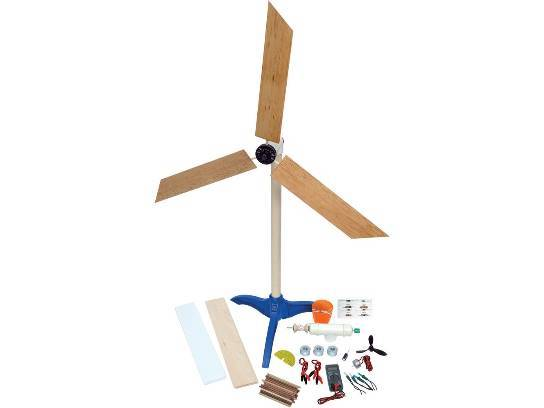Tested and approved by thousands of teachers worldwide, this is one of the most affordable and robust wind turbine kits on the market. Students can design turbine blades for it and test power output with a multimeter, LED lamps, or an ultra capacitor.

 /* styles */ Prefer to donate to a different Fund-a-Need item? Click HERE.
 table div table+table+table+table+table+table+table+table+table+table+table+table+table+table+table+table+table+table div table{width:100%;padding:0}table div table+table+table+table+table+table+table+table+table+table+table+table+table+table+table+table+table+table div table img{width:96.23%;padding:0;float:none}table div table+table+table+table+table+table+table+table+table+table+table+table+table+table+table+table+table+table div table td{width:100%;padding:0 1.88% 18px}/* styles */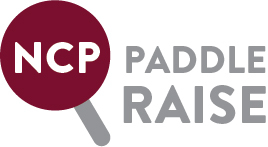Better Library, Brighter Future!

The NCP Library: the heart of the Northside community since 1998.

The library is the heart of NCP where students gather to study, collaborate, and socialize. Help us to modernize and revitalize the space for our motivated and talented students. Let’s provide our community with a library that meets the needs of our students in 2019 and beyond. Click HERE to hear from freshman Jake Philips and HERE to hear from sophomore Marco Salzetta.

We are happy to report that we have raised \$29,000 towards the library renovation so far. However, more funds are still needed to accomplish this massive undertaking that will transform the space.

Please make a donation to the NCP Fund for the Library today!

 table div table+table+table+table+table+table+table+table+table+table+table+table+table+table+table+table+table+table+table+table+table div table{width:100%;padding:0}table div table+table+table+table+table+table+table+table+table+table+table+table+table+table+table+table+table+table+table+table+table div table img{width:96.23%;padding:0;float:none}table div table+table+table+table+table+table+table+table+table+table+table+table+table+table+table+table+table+table+table+table+table div table td{width:100%;padding:0 1.88% 18px}/* styles */Parent Parties

Sign up to attend a party hosted by a Northside family. These parties are a great opportunity to meet and mingle with other Northside parents. We have something for everyone. Don't miss out, sign up today!

Moms Who Brunch

 table div table+table+table+table+table+table+table+table+table+table+table+table+table+table+table+table+table+table+table+table+table+table+table+table div table,table.module-23{width:45.66%;float:left;padding:0}table div table+table+table+table+table+table+table+table+table+table+table+table+table+table+table+table+table+table+table+table+table+table+table+table div table a{border:0 none;text-decoration:none}table div table+table+table+table+table+table+table+table+table+table+table+table+table+table+table+table+table+table+table+table+table+table+table+table div table img{width:100%!important;border:0 none;text-decoration:none}table div table+table+table+table+table+table+table+table+table+table+table+table+table+table+table+table+table+table+table+table+table+table+table+table div table td{width:100%;padding:0 20px 20px 0}/* styles */ Back again this year! Join us for delicious brunch items and cocktails. This party is a great opportunity to meet other Northside moms in a quiet, comfortable setting. Don't miss it! Sunday, June 2

Bourbon, Beers, & Burgers

 table div table+table+table+table+table+table+table+table+table+table+table+table+table+table+table+table+table+table+table+table+table+table+table+table+table+table div table,table.module-25{width:46.98%;float:left;padding:0}table div table+table+table+table+table+table+table+table+table+table+table+table+table+table+table+table+table+table+table+table+table+table+table+table+table+table div table a{border:0 none;text-decoration:none}table div table+table+table+table+table+table+table+table+table+table+table+table+table+table+table+table+table+table+table+table+table+table+table+table+table+table div table img{width:100%!important;border:0 none;text-decoration:none}table div table+table+table+table+table+table+table+table+table+table+table+table+table+table+table+table+table+table+table+table+table+table+table+table+table+table div table td{width:100%;padding:0 20px 20px 0}/* styles */ NEW THIS YEAR! Don't miss this casual celebratory night of Bourbon, Beers, and Burgers to mark the end of the school year. Saturday, June 22 at 7:00 pm.
 table div table+table+table+table+table+table+table+table+table+table+table+table+table+table+table+table+table+table+table+table+table+table+table+table+table+table+table+table div table{width:100%;padding:0}table div table+table+table+table+table+table+table+table+table+table+table+table+table+table+table+table+table+table+table+table+table+table+table+table+table+table+table+table div table img{width:96.23%;padding:0;float:none}table div table+table+table+table+table+table+table+table+table+table+table+table+table+table+table+table+table+table+table+table+table+table+table+table+table+table+table+table div table td{width:100%;padding:0 1.88% 18px}/* styles */Click HERE to see photos from the event.

 table div table+table+table+table+table+table+table+table+table+table+table+table+table+table+table+table+table+table+table+table+table+table+table+table+table+table+table+table+table+table div table{width:100%;padding:0}table div table+table+table+table+table+table+table+table+table+table+table+table+table+table+table+table+table+table+table+table+table+table+table+table+table+table+table+table+table+table div table img{width:96.23%;padding:0;float:none}table div table+table+table+table+table+table+table+table+table+table+table+table+table+table+table+table+table+table+table+table+table+table+table+table+table+table+table+table+table+table div table td{width:100%;padding:0 1.88% 18px}/* styles */This Week in Sports

Registration for spring sports is now open on the 8to18 website. Please be sure to register your students as soon as possible. Click HERE.

Women’s Lacrosse

▪ Monday, April 1, 5:00 pm (V) and 6:15 pm (JV) vs Lincoln Park at NCP
▪ Tuesday, April 2, 5:00 pm (V) and 6:15 pm (JV) vs Fenwick at Fenwick
▪ Thursday, April 4, 5:00 pm (V) and 6:15 pm (JV) vs Nazareth at NCP
▪ Friday, April 5, 4:30 pm (JV) vs Jones at NCP
▪ Saturday, April 6, 1:00 pm (V) and 2:15 pm (JV) vs Resurrection at Resurrection
 ▪ Monday, April 1, 5:00 pm (V) and 6:15 pm (JV) vs Lincoln Park at NCP
 ▪ Tuesday, April 2, 5:00 pm (V) and 6:15 pm (JV) vs Fenwick at Fenwick
 ▪ Thursday, April 4, 5:00 pm (V) and 6:15 pm (JV) vs Nazareth at NCP
 ▪ Friday, April 5, 4:30 pm (JV) vs Jones at NCP
 ▪ Saturday, April 6, 1:00 pm (V) and 2:15 pm (JV) vs Resurrection at Resurrection

Men’s Lacrosse

▪ Tuesday, April 2, TBA (V) v. Lincoln Park
▪ Thursday, April 4, TBA (V) v. Latin at Latin
 ▪ Tuesday, April 2, TBA (V) v. Lincoln Park
 ▪ Thursday, April 4, TBA (V) v. Latin at Latin

Men's Tennis

▪ Tuesday, April 2, 4:00 pm (V) v. Payton at Northside
 ▪ Tuesday, April 2, 4:00 pm (V) v. Payton at Northside

Women's Softball

▪ Monday, April 1, 4:30 pm (V & SO) v. Taft at Taft
▪ Wednesday, April 3, 4:30 pm (V & SO) v. Lincoln Park HS at Lincoln Park HS
 ▪ Monday, April 1, 4:30 pm (V & SO) v. Taft at Taft
 ▪ Wednesday, April 3, 4:30 pm (V & SO) v. Lincoln Park HS at Lincoln Park HS

Men's Baseball

▪ Monday, April 1, 4:30 pm vs Clemente at Clemente
▪ Wednesday, April 3, 4:30 pm vs Clemente at Rogers Park
▪ Friday, April 5, 4:30 pm vs Lane at Rogers Park
 ▪ Monday, April 1, 4:30 pm vs Clemente at Clemente
 ▪ Wednesday, April 3, 4:30 pm vs Clemente at Rogers Park
 ▪ Friday, April 5, 4:30 pm vs Lane at Rogers Park

Men’s Volleyball

▪ Monday, April 1, 5:00 pm (V) v. Steinmetz at Northside
▪ Wednesday, April 3, 5:00 pm (V) v. Schurz at Schurz
▪ Friday, April 5, 5:00 pm (V) v. York at Niles West Invitational
▪ Saturday, April 6, 9:00 am (V) at Niles West Invitational
 ▪ Monday, April 1, 5:00 pm (V) v. Steinmetz at Northside
 ▪ Wednesday, April 3, 5:00 pm (V) v. Schurz at Schurz
 ▪ Friday, April 5, 5:00 pm (V) v. York at Niles West Invitational
 ▪ Saturday, April 6, 9:00 am (V) at Niles West Invitational

Women's Water Polo

▪ Monday, April 1, 5:00 pm v. Schaumburg at Schaumburg
 ▪ Monday, April 1, 5:00 pm v. Schaumburg at Schaumburg

Men's Water Polo

▪ Tuesday, April 2, 5:00 pm v. Whitney Young at Northside
▪ Wednesday, April 3, 6:00 pm v. Taft at Northside
 ▪ Tuesday, April 2, 5:00 pm v. Whitney Young at Northside
 ▪ Wednesday, April 3, 6:00 pm v. Taft at Northside

Men's Outdoor Track & Field

▪ Saturday, April 6, 9:00 am, meet at Niles West
 ▪ Saturday, April 6, 9:00 am, meet at Niles West
 table div table+table+table+table+table+table+table+table+table+table+table+table+table+table+table+table+table+table+table+table+table+table+table+table+table+table+table+table+table+table+table+table div table{width:100%;padding:0}table div table+table+table+table+table+table+table+table+table+table+table+table+table+table+table+table+table+table+table+table+table+table+table+table+table+table+table+table+table+table+table+table div table img{width:96.23%;padding:0;float:none}table div table+table+table+table+table+table+table+table+table+table+table+table+table+table+table+table+table+table+table+table+table+table+table+table+table+table+table+table+table+table+table+table div table td{width:100%;padding:0 1.88% 18px}/* styles */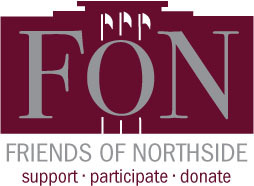Give the Gift of Your Time and Talents

Become a FON Board Member

Friends of Northside (FON) is a parent organization that works to support the Northside community through fundraising and volunteerism. All parents and guardians are automatically members of FON. Board positions are available for those who would like to take a more active role.

FON's fundraising seeks to raise money with the goals of replacing lost funding from CPS, supporting the school’s mission, and maintaining and enhancing the learning experience for all NCP students. FON efforts include the Annual Appeal, Spring Benefit, and Cubby Walk Walkathon, among others.

FON also provides volunteers and hospitality to the NCP community. It plans social/educational programs, recruits volunteers for various NCP events such as Open House, distributes this weekly email communication, and maintains the student directory.

Come see what we're all about!

Join us at our next meeting and learn more about what's happening at Northside and how you can make an impact.

Tuesday, April 23, 7:00 pm
Faculty Lounge

 table div table+table+table+table+table+table+table+table+table+table+table+table+table+table+table+table+table+table+table+table+table+table+table+table+table+table+table+table+table+table+table+table+table+table div table{width:100%;padding:0}table div table+table+table+table+table+table+table+table+table+table+table+table+table+table+table+table+table+table+table+table+table+table+table+table+table+table+table+table+table+table+table+table+table+table div table img{width:96.23%;padding:0;float:none}table div table+table+table+table+table+table+table+table+table+table+table+table+table+table+table+table+table+table+table+table+table+table+table+table+table+table+table+table+table+table+table+table+table+table div table td{width:100%;padding:0 1.88% 18px}/* styles */On-line registration for Parent-Teacher conferences will be open from Sunday, March 31, until midnight Monday, April 8. You will have the opportunity to access teachers’ calendars and schedule a convenient conference time.

Click HERE for instructions and the HERE for the online sign-up.

 table div table+table+table+table+table+table+table+table+table+table+table+table+table+table+table+table+table+table+table+table+table+table+table+table+table+table+table+table+table+table+table+table+table+table+table+table div table{width:100%;padding:0}table div table+table+table+table+table+table+table+table+table+table+table+table+table+table+table+table+table+table+table+table+table+table+table+table+table+table+table+table+table+table+table+table+table+table+table+table div table img{width:96.23%;padding:0;float:none}table div table+table+table+table+table+table+table+table+table+table+table+table+table+table+table+table+table+table+table+table+table+table+table+table+table+table+table+table+table+table+table+table+table+table+table+table div table td{width:100%;padding:0 1.88% 18px}/* styles */Students can Attend the Chicago Latino Film Festival for Supplemental Credit in Spanish Class

Señor Blease wanted to let you know about a wonderful opportunity to receive supplemental credit for your child in his Spanish class. He has encouraged students to go to the Chicago Latino Film Festival for the past 18 years, and they have loved it. Parents should know that although this experience is amazing and it is a celebration of international films and culture, some films may not be appropriate for some people.

He encourages you to take a look at THIS website to read the descriptions of movies students can see so you can determine which movies would be more appropriate for your child.

The festival begins March 28 and goes until April 11. The cost for students is \$10 with ID., but he will be exploring less expensive options, and he will post them to his website if he finds them. Thank you for your support of the Spanish program! If you have any questions, please contact Señor Blease at rblease@cps.edu.

 table div table+table+table+table+table+table+table+table+table+table+table+table+table+table+table+table+table+table+table+table+table+table+table+table+table+table+table+table+table+table+table+table+table+table+table+table+table+table div table{width:100%;padding:0}table div table+table+table+table+table+table+table+table+table+table+table+table+table+table+table+table+table+table+table+table+table+table+table+table+table+table+table+table+table+table+table+table+table+table+table+table+table+table div table img{width:96.23%;padding:0;float:none}table div table+table+table+table+table+table+table+table+table+table+table+table+table+table+table+table+table+table+table+table+table+table+table+table+table+table+table+table+table+table+table+table+table+table+table+table+table+table div table td{width:100%;padding:0 1.88% 18px}/* styles */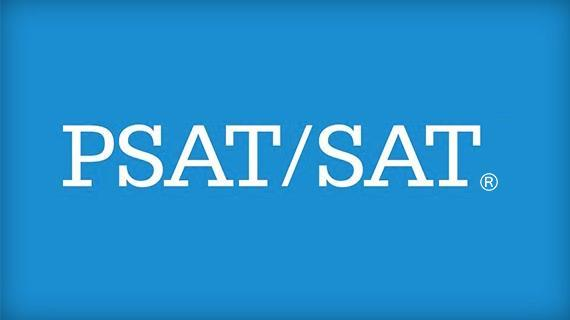Spring Testing 2019 - SAT/PSAT

During the week of April 8, students in grades 9-11 will participate in city and statewide testing of the SAT/PSAT.

Tuesday, April 9
The SAT will be administered to 11th grade students.

Attendance day for 11th graders only; non-attendance day for 9th, 10th, and 12th graders

Wednesday, April 10
The PSAT will be administered to 9th and 10th grade students.

Attendance day for 9th and 10th graders only; non-attendance day for 11th and 12th graders

For 11th grade and 12th grade, there will be a series of optional college visits and service projects. Students will be asked to confirm participation.

 table div table+table+table+table+table+table+table+table+table+table+table+table+table+table+table+table+table+table+table+table+table+table+table+table+table+table+table+table+table+table+table+table+table+table+table+table+table+table+table+table div table{width:100%;padding:0}table div table+table+table+table+table+table+table+table+table+table+table+table+table+table+table+table+table+table+table+table+table+table+table+table+table+table+table+table+table+table+table+table+table+table+table+table+table+table+table+table div table img{width:96.23%;padding:0;float:none}table div table+table+table+table+table+table+table+table+table+table+table+table+table+table+table+table+table+table+table+table+table+table+table+table+table+table+table+table+table+table+table+table+table+table+table+table+table+table+table+table div table td{width:100%;padding:0 1.88% 18px}/* styles */Important Dates to Note in April

Thursday, April 4 -- End of Quarter 3
Friday, April 5 -- No Classes - School Improvement Day
Tuesday, April 9 -- SAT testing for juniors, non-attendance day for freshmen, sophomores and seniors
Wednesday, April 10 -- PSAT testing for freshmen and sophomores; optional day of service for juniors and seniors
Wednesday, April 10 -- 7:00 pm Athletic Boosters Meeting
Thursday, April 11 -- Parent-Teacher Conferences - Non-attendance day for students
April 15 through April 19 -- Spring Break
Tuesday, April 23 -- 6:30 pm LSC Meeting; 7:00 pm Friends of Northside Meeting
Thursday, April 25 -- Cubby Walk
Thursday, April 25 -- 7:00 pm Spring Concert

 table div table+table+table+table+table+table+table+table+table+table+table+table+table+table+table+table+table+table+table+table+table+table+table+table+table+table+table+table+table+table+table+table+table+table+table+table+table+table+table+table+table+table div table{width:100%;padding:0}table div table+table+table+table+table+table+table+table+table+table+table+table+table+table+table+table+table+table+table+table+table+table+table+table+table+table+table+table+table+table+table+table+table+table+table+table+table+table+table+table+table+table div table img{width:96.23%;padding:0;float:none}table div table+table+table+table+table+table+table+table+table+table+table+table+table+table+table+table+table+table+table+table+table+table+table+table+table+table+table+table+table+table+table+table+table+table+table+table+table+table+table+table+table+table div table td{width:100%;padding:0 1.88% 18px}/* styles */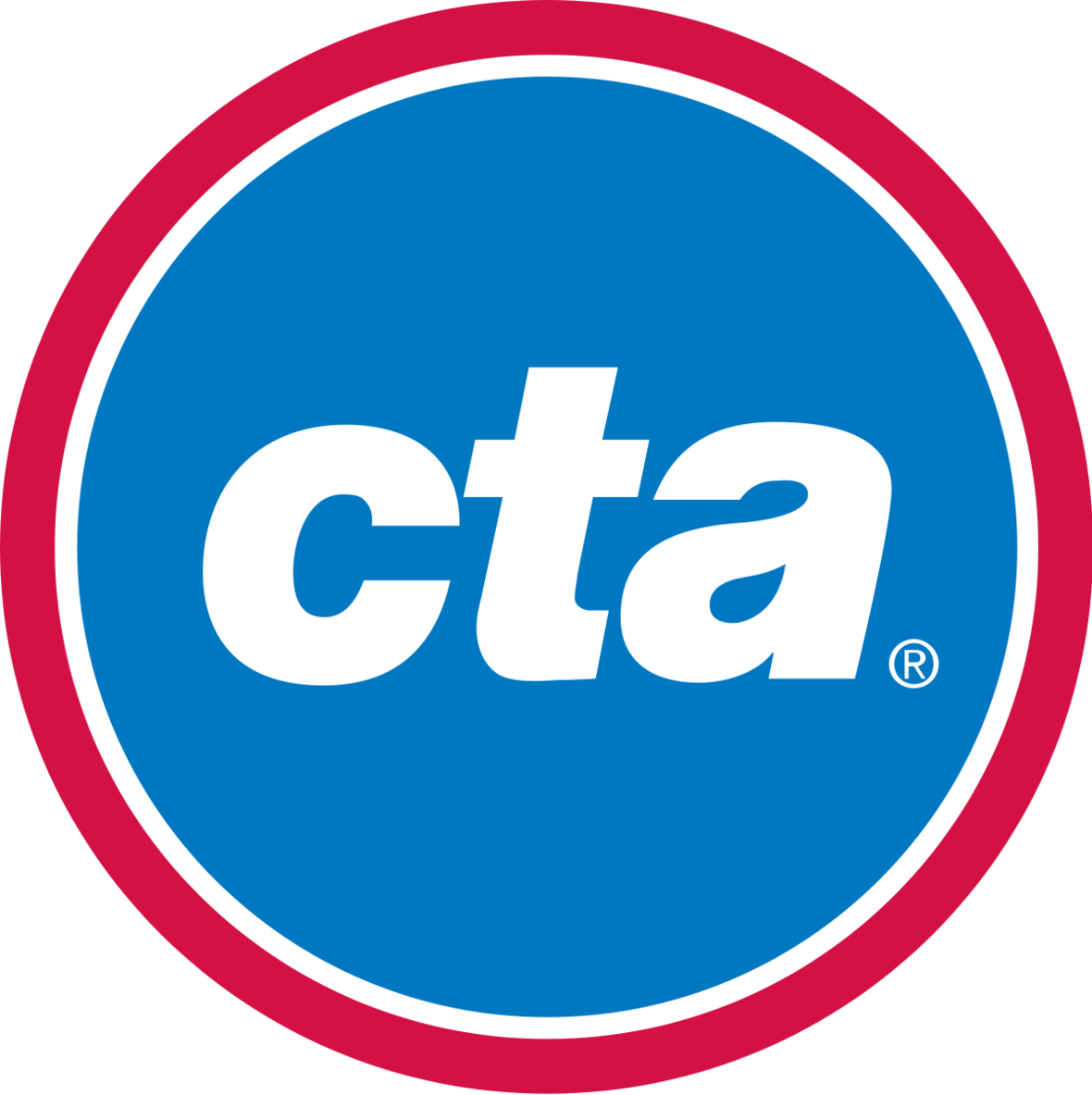CTA Safety Presentation

Wednesday, May 22

A representative from CTA will present to NCP students about navigating and staying safe on public transportation. Please encourage your students to learn about the support and safety tips offered to ensure an enjoyable commute to and from school.

 table div table+table+table+table+table+table+table+table+table+table+table+table+table+table+table+table+table+table+table+table+table+table+table+table+table+table+table+table+table+table+table+table+table+table+table+table+table+table+table+table+table+table+table+table div table{width:100%;padding:0}table div table+table+table+table+table+table+table+table+table+table+table+table+table+table+table+table+table+table+table+table+table+table+table+table+table+table+table+table+table+table+table+table+table+table+table+table+table+table+table+table+table+table+table+table div table img{width:96.23%;padding:0;float:none}table div table+table+table+table+table+table+table+table+table+table+table+table+table+table+table+table+table+table+table+table+table+table+table+table+table+table+table+table+table+table+table+table+table+table+table+table+table+table+table+table+table+table+table+table div table td{width:100%;padding:0 1.88% 18px}/* styles */Friends Of Northside Speaker Series

PRESENTS

May 23, 2019, 6:30 pm

FEATURING…

Dr. Debra Kissen

Dr. Debra Kissen is the Owner and Clinical Director of the Light On Anxiety Treatment Center, www.lightonanxiety.com. She specializes in Cognitive Behavioral Therapy (CBT) for anxiety and related disorders, is the author of the Panic Workbook for Teens and is an active contributor to the Huffington Post. Dr. Kissen will provide tips and strategies to assist with parenting young adults and share:

▪ How to tell the difference between every day stress and anxiety
▪ How to model effective coping skills for your teen
▪ How to compassionately push your child forward to assist them in facing their fears
 ▪ How to tell the difference between every day stress and anxiety
 ▪ How to model effective coping skills for your teen
 ▪ How to compassionately push your child forward to assist them in facing their fears
 /* styles */ Have a question you would like to submit anonymously in advance? Email it to julie@lightonanxiety.com with the Subject line marked “NCP Parent Speaker Series”. Dr. Kissen will do her best to address these during her presentation. Look for additional details soon in the Northside Parents Facebook Page and at FriendsofNorthside.org
 table div table+table+table+table+table+table+table+table+table+table+table+table+table+table+table+table+table+table+table+table+table+table+table+table+table+table+table+table+table+table+table+table+table+table+table+table+table+table+table+table+table+table+table+table+table+table+table+table div table{width:100%;padding:0}table div table+table+table+table+table+table+table+table+table+table+table+table+table+table+table+table+table+table+table+table+table+table+table+table+table+table+table+table+table+table+table+table+table+table+table+table+table+table+table+table+table+table+table+table+table+table+table+table div table img{width:96.23%;padding:0;float:none}table div table+table+table+table+table+table+table+table+table+table+table+table+table+table+table+table+table+table+table+table+table+table+table+table+table+table+table+table+table+table+table+table+table+table+table+table+table+table+table+table+table+table+table+table+table+table+table+table div table td{width:100%;padding:0 1.88% 18px}/* styles */Juniors - Class of 2020! Save the Dates!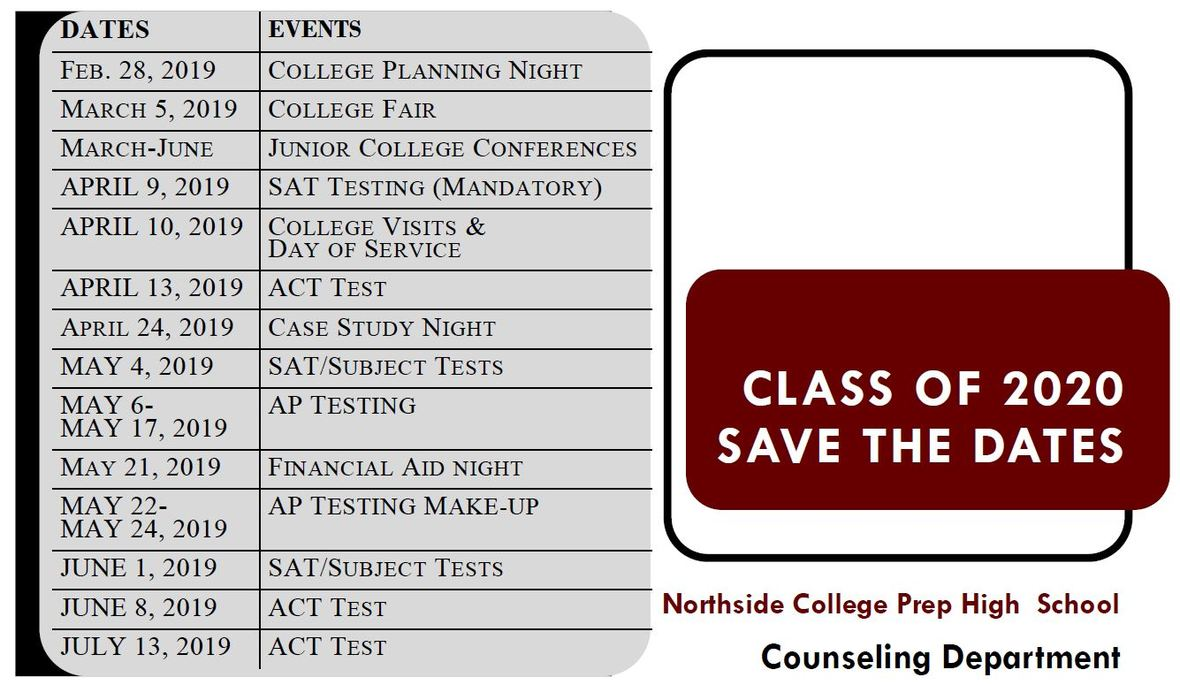table div table+table+table+table+table+table+table+table+table+table+table+table+table+table+table+table+table+table+table+table+table+table+table+table+table+table+table+table+table+table+table+table+table+table+table+table+table+table+table+table+table+table+table+table+table+table+table+table+table+table+table div table{width:100%;padding:0}table div table+table+table+table+table+table+table+table+table+table+table+table+table+table+table+table+table+table+table+table+table+table+table+table+table+table+table+table+table+table+table+table+table+table+table+table+table+table+table+table+table+table+table+table+table+table+table+table+table+table+table div table img{width:96.23%;padding:0;float:none}table div table+table+table+table+table+table+table+table+table+table+table+table+table+table+table+table+table+table+table+table+table+table+table+table+table+table+table+table+table+table+table+table+table+table+table+table+table+table+table+table+table+table+table+table+table+table+table+table+table+table+table div table td{width:100%;padding:0 1.88% 18px}/* styles */Travel this Spring with Northside

Spring Break 2019 Trip to the Grand Canyon

Students will have a chance to connect with the majesty of our National Park system. We will spend 5 nights in the Grand Canyon camping, hiking, stargazing, and learning wilderness skills. Students will participate in a second-semester colloquium to prepare them for the trip. Trip sponsors and chaperones include Ms. Qazi, Ms. Schaldenbrand, and Mr. Barry Smith. Approximate cost: \$1,000 (includes flight, food, and lodging).

 table div table+table+table+table+table+table+table+table+table+table+table+table+table+table+table+table+table+table+table+table+table+table+table+table+table+table+table+table+table+table+table+table+table+table+table+table+table+table+table+table+table+table+table+table+table+table+table+table+table+table+table+table+table div table{width:100%;padding:0}table div table+table+table+table+table+table+table+table+table+table+table+table+table+table+table+table+table+table+table+table+table+table+table+table+table+table+table+table+table+table+table+table+table+table+table+table+table+table+table+table+table+table+table+table+table+table+table+table+table+table+table+table+table div table img{width:96.23%;padding:0;float:none}table div table+table+table+table+table+table+table+table+table+table+table+table+table+table+table+table+table+table+table+table+table+table+table+table+table+table+table+table+table+table+table+table+table+table+table+table+table+table+table+table+table+table+table+table+table+table+table+table+table+table+table+table+table div table td{width:100%;padding:0 1.88% 18px}/* styles */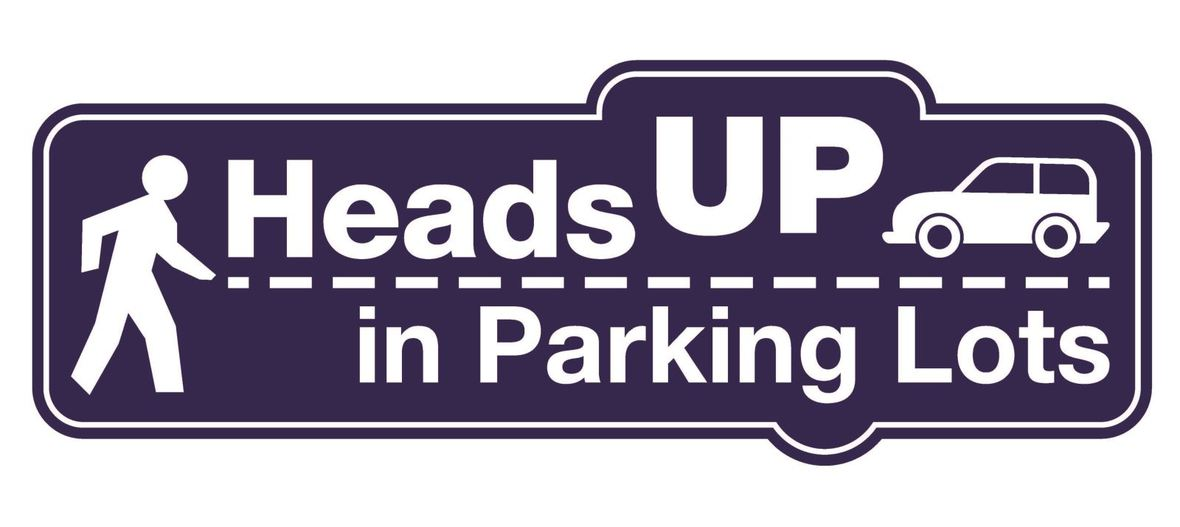Parking Lot Safety and Procedures

Our NCP school parking lot is a busy place before and after school. Families dropping off and picking up students are asked to be mindful of both safety and courtesy. Please assist us by ensuring that traffic lanes stay clear during drop-off and pick-up times. Pulling over to the curb on the right as your student enters or exits your vehicle keeps students safe and traffic flow efficient.

Please remember that ALL VEHICLES MUST EXIT ONTO KEDZIE. You may enter the parking lot from Kedzie or Bryn Mawr, but regardless of where you enter, all cars must exit onto Kedzie. For the safety of our students and the rest of the Northside community, please do not exit onto Bryn Mawr.

 table div table+table+table+table+table+table+table+table+table+table+table+table+table+table+table+table+table+table+table+table+table+table+table+table+table+table+table+table+table+table+table+table+table+table+table+table+table+table+table+table+table+table+table+table+table+table+table+table+table+table+table+table+table+table+table+table div table{width:100%;padding:0}table div table+table+table+table+table+table+table+table+table+table+table+table+table+table+table+table+table+table+table+table+table+table+table+table+table+table+table+table+table+table+table+table+table+table+table+table+table+table+table+table+table+table+table+table+table+table+table+table+table+table+table+table+table+table+table+table div table img{width:96.23%;padding:0;float:none}table div table+table+table+table+table+table+table+table+table+table+table+table+table+table+table+table+table+table+table+table+table+table+table+table+table+table+table+table+table+table+table+table+table+table+table+table+table+table+table+table+table+table+table+table+table+table+table+table+table+table+table+table+table+table+table+table div table td{width:100%;padding:0 1.88% 18px}/* styles */CPS Volunteer Requirements

According to CPS policy, anybody who is going to be in contact with students must be approved as a CPS volunteer. There are two levels of volunteers, Level 1 and Level 2.

Level II Volunteers

(This process can be completed in a day & is required even of volunteers for Friends of Northside events)
A fingerprint criminal background check is not required for Level II Volunteers.

A Level II Volunteer is:

1. A parent providing supervised volunteer service in their child’s school or classroom for less than 10 hours/week;
2. Any individual providing volunteer service for less than 5 hours/week at a school where s/he does not have a child enrolled;
3. An individual providing incidental volunteer service with no ongoing individualized interaction with a student(s) including those who speak at a class/assembly, judge academic competitions, give musical performance, participate in the “Principal for a Day” program, job shadowing event or other one-time event provided where there is direct supervision of the activity/event by regular school employees;
4. A parent accompanying his/her child’s class on a one-day field trip or another type of extracurricular activity that does not involve an overnight stay; and/or
5. An individual providing volunteer service on projects/activities involving no or nominal contact with children (home-based volunteer activities, volunteers serving at Central or Network office).
 1 A parent providing supervised volunteer service in their child’s school or classroom for less than 10 hours/week;
 2 Any individual providing volunteer service for less than 5 hours/week at a school where s/he does not have a child enrolled;
 3 An individual providing incidental volunteer service with no ongoing individualized interaction with a student(s) including those who speak at a class/assembly, judge academic competitions, give musical performance, participate in the “Principal for a Day” program, job shadowing event or other one-time event provided where there is direct supervision of the activity/event by regular school employees;
 4 A parent accompanying his/her child’s class on a one-day field trip or another type of extracurricular activity that does not involve an overnight stay; and/or
 5 An individual providing volunteer service on projects/activities involving no or nominal contact with children (home-based volunteer activities, volunteers serving at Central or Network office).

Level I Volunteers

(Please allow 8-12 weeks for the entire process)
A fingerprint criminal background check is required for all Level I Volunteers.

A Level I volunteer is:

1. A parent providing volunteer service in their child’s school or classroom for 10 or more hours/week;
2. An individual providing volunteer service for 5 or more hours/week at a school where s/he does not have a child enrolled; and/or
3. Any individual chaperoning an overnight school-sponsored trip, regardless of the hours/week that the volunteer serves (See also the Student Travel Policy).
 1 A parent providing volunteer service in their child’s school or classroom for 10 or more hours/week;
 2 An individual providing volunteer service for 5 or more hours/week at a school where s/he does not have a child enrolled; and/or
 3 Any individual chaperoning an overnight school-sponsored trip, regardless of the hours/week that the volunteer serves (See also the Student Travel Policy).

To become a volunteer, please visit CPS' Volunteer website and follow the instructions. There is also a link on Northside's website on the Parents page Northside Volunteers.

Assistant Principal Kaitlin O'Hare is Northside's Volunteer Coordinator. After applying to become a volunteer, you must visit the school and show Ms. O'Hare your ID, so she can approve you to move forward in the volunteer process. For Level II volunteers, that is the only step you must take. For Level I, you will receive further instructions from CPS.

 table div table+table+table+table+table+table+table+table+table+table+table+table+table+table+table+table+table+table+table+table+table+table+table+table+table+table+table+table+table+table+table+table+table+table+table+table+table+table+table+table+table+table+table+table+table+table+table+table+table+table+table+table+table+table+table+table+table+table div table{width:100%;padding:0}table div table+table+table+table+table+table+table+table+table+table+table+table+table+table+table+table+table+table+table+table+table+table+table+table+table+table+table+table+table+table+table+table+table+table+table+table+table+table+table+table+table+table+table+table+table+table+table+table+table+table+table+table+table+table+table+table+table+table div table img{width:96.23%;padding:0;float:none}table div table+table+table+table+table+table+table+table+table+table+table+table+table+table+table+table+table+table+table+table+table+table+table+table+table+table+table+table+table+table+table+table+table+table+table+table+table+table+table+table+table+table+table+table+table+table+table+table+table+table+table+table+table+table+table+table+table+table div table td{width:100%;padding:0 1.88% 18px}/* styles */table.module-58{width:94.34%;padding:0}table div table+table+table+table+table+table+table+table+table+table+table+table+table+table+table+table+table+table+table+table+table+table+table+table+table+table+table+table+table+table+table+table+table+table+table+table+table+table+table+table+table+table+table+table+table+table+table+table+table+table+table+table+table+table+table+table+table+table+table div table{width:94.34%;float:none;margin-left:auto;margin-right:auto;padding:0}table div table+table+table+table+table+table+table+table+table+table+table+table+table+table+table+table+table+table+table+table+table+table+table+table+table+table+table+table+table+table+table+table+table+table+table+table+table+table+table+table+table+table+table+table+table+table+table+table+table+table+table+table+table+table+table+table+table+table+table div table a{border:0 none;text-decoration:none}table div table+table+table+table+table+table+table+table+table+table+table+table+table+table+table+table+table+table+table+table+table+table+table+table+table+table+table+table+table+table+table+table+table+table+table+table+table+table+table+table+table+table+table+table+table+table+table+table+table+table+table+table+table+table+table+table+table+table+table div table img{width:100%!important;border:0 none;text-decoration:none}table div table+table+table+table+table+table+table+table+table+table+table+table+table+table+table+table+table+table+table+table+table+table+table+table+table+table+table+table+table+table+table+table+table+table+table+table+table+table+table+table+table+table+table+table+table+table+table+table+table+table+table+table+table+table+table+table+table+table+table div table td{width:100%;padding:0}/* styles */
 /* styles */ Check out The HoofBeat, Northside's online newspaper, to get NCP news from the student perspective!
 table div table+table+table+table+table+table+table+table+table+table+table+table+table+table+table+table+table+table+table+table+table+table+table+table+table+table+table+table+table+table+table+table+table+table+table+table+table+table+table+table+table+table+table+table+table+table+table+table+table+table+table+table+table+table+table+table+table+table+table+table+table div table{width:100%;padding:0}table div table+table+table+table+table+table+table+table+table+table+table+table+table+table+table+table+table+table+table+table+table+table+table+table+table+table+table+table+table+table+table+table+table+table+table+table+table+table+table+table+table+table+table+table+table+table+table+table+table+table+table+table+table+table+table+table+table+table+table+table+table div table img{width:96.23%;padding:0;float:none}table div table+table+table+table+table+table+table+table+table+table+table+table+table+table+table+table+table+table+table+table+table+table+table+table+table+table+table+table+table+table+table+table+table+table+table+table+table+table+table+table+table+table+table+table+table+table+table+table+table+table+table+table+table+table+table+table+table+table+table+table+table div table td{width:100%;padding:0 1.88% 18px}/* styles */table.module-61{width:57.17%;padding:0}table div table+table+table+table+table+table+table+table+table+table+table+table+table+table+table+table+table+table+table+table+table+table+table+table+table+table+table+table+table+table+table+table+table+table+table+table+table+table+table+table+table+table+table+table+table+table+table+table+table+table+table+table+table+table+table+table+table+table+table+table+table+table div table{width:57.17%;float:none;margin-left:auto;margin-right:auto;padding:0}table div table+table+table+table+table+table+table+table+table+table+table+table+table+table+table+table+table+table+table+table+table+table+table+table+table+table+table+table+table+table+table+table+table+table+table+table+table+table+table+table+table+table+table+table+table+table+table+table+table+table+table+table+table+table+table+table+table+table+table+table+table+table div table a{border:0 none;text-decoration:none}table div table+table+table+table+table+table+table+table+table+table+table+table+table+table+table+table+table+table+table+table+table+table+table+table+table+table+table+table+table+table+table+table+table+table+table+table+table+table+table+table+table+table+table+table+table+table+table+table+table+table+table+table+table+table+table+table+table+table+table+table+table+table div table img{width:100%!important;border:0 none;text-decoration:none}table div table+table+table+table+table+table+table+table+table+table+table+table+table+table+table+table+table+table+table+table+table+table+table+table+table+table+table+table+table+table+table+table+table+table+table+table+table+table+table+table+table+table+table+table+table+table+table+table+table+table+table+table+table+table+table+table+table+table+table+table+table+table div table td{width:100%;padding:0}/* styles */

What is FAN?

FAN is Family Action Network, an organization which connects parents, educators, and professionals through collaborative programming that educates, inspires, and positively impacts the broader community. Nearly every week during the school year there will be a dynamic and important speaker, discussing the issues that interest you.

FAN events are free to all.

 table div table+table+table+table+table+table+table+table+table+table+table+table+table+table+table+table+table+table+table+table+table+table+table+table+table+table+table+table+table+table+table+table+table+table+table+table+table+table+table+table+table+table+table+table+table+table+table+table+table+table+table+table+table+table+table+table+table+table+table+table+table+table+table+table div table{width:100%;padding:0}table div table+table+table+table+table+table+table+table+table+table+table+table+table+table+table+table+table+table+table+table+table+table+table+table+table+table+table+table+table+table+table+table+table+table+table+table+table+table+table+table+table+table+table+table+table+table+table+table+table+table+table+table+table+table+table+table+table+table+table+table+table+table+table+table div table img{width:96.23%;padding:0;float:none}table div table+table+table+table+table+table+table+table+table+table+table+table+table+table+table+table+table+table+table+table+table+table+table+table+table+table+table+table+table+table+table+table+table+table+table+table+table+table+table+table+table+table+table+table+table+table+table+table+table+table+table+table+table+table+table+table+table+table+table+table+table+table+table+table div table td{width:100%;padding:0 1.88% 18px}/* styles */Do you have news or team results to share with the Northside parent community?

 table div table+table+table+table+table+table+table+table+table+table+table+table+table+table+table+table+table+table+table+table+table+table+table+table+table+table+table+table+table+table+table+table+table+table+table+table+table+table+table+table+table+table+table+table+table+table+table+table+table+table+table+table+table+table+table+table+table+table+table+table+table+table+table+table+table+table+table div table{width:100%;padding:0}table div table+table+table+table+table+table+table+table+table+table+table+table+table+table+table+table+table+table+table+table+table+table+table+table+table+table+table+table+table+table+table+table+table+table+table+table+table+table+table+table+table+table+table+table+table+table+table+table+table+table+table+table+table+table+table+table+table+table+table+table+table+table+table+table+table+table+table div table img{width:96.23%;padding:0;float:none}table div table+table+table+table+table+table+table+table+table+table+table+table+table+table+table+table+table+table+table+table+table+table+table+table+table+table+table+table+table+table+table+table+table+table+table+table+table+table+table+table+table+table+table+table+table+table+table+table+table+table+table+table+table+table+table+table+table+table+table+table+table+table+table+table+table+table+table div table td{width:100%;padding:0 1.88% 18px}/* styles */FON Connect

Friends of Northside uses a customer/constituent relationship management (CRM) system known as FON Connect that allows our parent volunteer organizations to work from one database.

FON Connect serves as the NCP Family Directory and allows users to review their donation history, update their contact information, register for upcoming events, and more. Each parent has a separate account. Transactions, including donations, are recorded in the account of the parent who initiated the transaction or donation.

If you’re new to FON Connect, you'll need to set up a login and password. To do so:

▪ Click on “Forgot Password?”
▪ Check your email for the link to create a login name and password.
 ▪ Click on “Forgot Password?”
 ▪ Check your email for the link to create a login name and password.

Once you have set up your FON Connect account, keep your login and password in a safe, convenient place because they will be your keys to the database throughout your years at Northside.

 table div table+table+table+table+table+table+table+table+table+table+table+table+table+table+table+table+table+table+table+table+table+table+table+table+table+table+table+table+table+table+table+table+table+table+table+table+table+table+table+table+table+table+table+table+table+table+table+table+table+table+table+table+table+table+table+table+table+table+table+table+table+table+table+table+table+table+table+table+table div table{width:100%;padding:0}table div table+table+table+table+table+table+table+table+table+table+table+table+table+table+table+table+table+table+table+table+table+table+table+table+table+table+table+table+table+table+table+table+table+table+table+table+table+table+table+table+table+table+table+table+table+table+table+table+table+table+table+table+table+table+table+table+table+table+table+table+table+table+table+table+table+table+table+table+table div table img{width:96.23%;padding:0;float:none}table div table+table+table+table+table+table+table+table+table+table+table+table+table+table+table+table+table+table+table+table+table+table+table+table+table+table+table+table+table+table+table+table+table+table+table+table+table+table+table+table+table+table+table+table+table+table+table+table+table+table+table+table+table+table+table+table+table+table+table+table+table+table+table+table+table+table+table+table+table div table td{width:100%;padding:0 1.88% 18px}/* styles */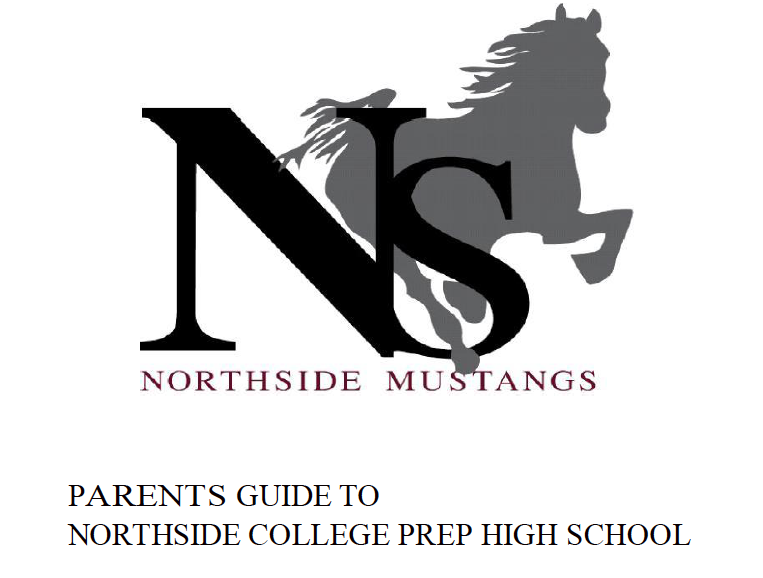Navigating Northside

Navigating Northside, our parent handbook, is available on the school website under the Parents tab. Click HERE to access it directly.

 table div table+table+table+table+table+table+table+table+table+table+table+table+table+table+table+table+table+table+table+table+table+table+table+table+table+table+table+table+table+table+table+table+table+table+table+table+table+table+table+table+table+table+table+table+table+table+table+table+table+table+table+table+table+table+table+table+table+table+table+table+table+table+table+table+table+table+table+table+table+table+table div table{width:100%;padding:0}table div table+table+table+table+table+table+table+table+table+table+table+table+table+table+table+table+table+table+table+table+table+table+table+table+table+table+table+table+table+table+table+table+table+table+table+table+table+table+table+table+table+table+table+table+table+table+table+table+table+table+table+table+table+table+table+table+table+table+table+table+table+table+table+table+table+table+table+table+table+table+table div table img{width:96.23%;padding:0;float:none}table div table+table+table+table+table+table+table+table+table+table+table+table+table+table+table+table+table+table+table+table+table+table+table+table+table+table+table+table+table+table+table+table+table+table+table+table+table+table+table+table+table+table+table+table+table+table+table+table+table+table+table+table+table+table+table+table+table+table+table+table+table+table+table+table+table+table+table+table+table+table+table div table td{width:100%;padding:0 1.88% 18px}/* styles */College Planning Night

Junior Students and Parents,

Thank you for attending the Northside Prep, Junior College Planning Night on Thursday, March 14. The counseling team is looking forward to discussing your student's post-secondary plans during the upcoming College Conferences. For more details about scheduling your college conference contact your respective college counselor. To schedule a college conference with Ms. Medina, please contact Ms. Moore at mcmoore6@cps.edu.

CPS Scholarships and Non-NCP Events

For more information about general CPS scholarships and post-secondary events outside of Northside College Prep feel free to visit the NCP College Counseling Calendar HERE.

SAT Prep Course

Wednesdays through April 3, 2:00 - 5:00 pm
Bring sharpened pencils and a calculator for every class.

For more information, contact Monique Moore, Northside Prep College Counselor at mcmoore6@cps.edu or (773) 524-3954 x26811.Naviance CCLR Live by Kaplan

College, Career, and Life ReadinessNCP College Planning Handbook

This is a great resource that guides students through the entire college process. Click HERE to access a copy.

NCP College Planning Additional Resources

Click on the links below to check out the additional resources on the Northside College Prep website:

 table div table+table+table+table+table+table+table+table+table+table+table+table+table+table+table+table+table+table+table+table+table+table+table+table+table+table+table+table+table+table+table+table+table+table+table+table+table+table+table+table+table+table+table+table+table+table+table+table+table+table+table+table+table+table+table+table+table+table+table+table+table+table+table+table+table+table+table+table+table+table+table+table+table+table+table+table+table+table+table div table{width:100%;padding:0}table div table+table+table+table+table+table+table+table+table+table+table+table+table+table+table+table+table+table+table+table+table+table+table+table+table+table+table+table+table+table+table+table+table+table+table+table+table+table+table+table+table+table+table+table+table+table+table+table+table+table+table+table+table+table+table+table+table+table+table+table+table+table+table+table+table+table+table+table+table+table+table+table+table+table+table+table+table+table+table div table img{width:96.23%;padding:0;float:none}table div table+table+table+table+table+table+table+table+table+table+table+table+table+table+table+table+table+table+table+table+table+table+table+table+table+table+table+table+table+table+table+table+table+table+table+table+table+table+table+table+table+table+table+table+table+table+table+table+table+table+table+table+table+table+table+table+table+table+table+table+table+table+table+table+table+table+table+table+table+table+table+table+table+table+table+table+table+table+table div table td{width:100%;padding:0 1.88% 18px}/* styles */Thank You to Our Individual and Corporate Sponsors for the 2019 Spring Benefit

Friends of Northside and the 2019 Spring Benefit Committee would like to thank our Individual and Corporate Sponsors whose support was critical to making the event a financial success.

▪ Kristen & Paul Collier
▪ Julie Trester & Nick Newgarden
▪ Carol Anne & Bart Huff
▪ Jim Kosciolek & Jeanine O'Brien
▪ Janice Cody & Allen Wall
▪ Myra Wagner & Glen Spear
▪ Debra & Stephen Swan

▪ Caryn & Charles Brissman
▪ Janice Maxwell & Kevin Carter
▪ Anne Marie Piazza-Cook & Rafael Cook
▪ Pamela Peters & Michael Hughes
▪ Dawn Bartholomew & Todd Maxwell
▪ Wendy & Matt Phillips
▪ Kim & George Ruhana
▪ Meg & Paul Salzetta
▪ Susan & John Schaefer
▪ Michele & Steven Sparks
▪ Elizabeth & George Phillips-Sorich

▪ Izzi & Scott Baskin
▪ Kim Chhay & Edward Huang
▪ Pinki & Nehal Desai
▪ Jennifer & Tim Devine
▪ Holly & Paul Edlund
▪ Matthew & Lisa Farmer
▪ Anna Florek & Brian Carlson
▪ Dawn Zarembski & Mark Franke
▪ Nancy Liljedahl & Joel Friedman
▪ Linda & Ed Graziano
▪ Kimberly Chase & Joshua Harding
▪ Patricia Bayerlein & Michael Hoffman
▪ Kirsten Brandt & Steve Kibler
▪ Dianne & Thomas Malueg
▪ Kelly & Eric Mest
▪ Kamaljeet & Karna Murthy
▪ Art & Susan Nolan
▪ Ony Achufusi & Marta Olah
▪ Maria Maldonado & Esau Orduno
▪ Erin Menninger Klemm & Kurt Klemm
▪ Caroline & Ram Padmanabhan
▪ Lorna Propes & Ronald Himel
▪ Karen & Fabian Sanchez
▪ Matthew & Erika Schechter
▪ Irma & Peter Sodini
▪ Anne Suh & Alan Hauser
▪ Elizabeth & Brent Vincent
▪ Kelly & Michael Zehfusstable div table+table+table+table+table+table+table+table+table+table+table+table+table+table+table+table+table+table+table+table+table+table+table+table+table+table+table+table+table+table+table+table+table+table+table+table+table+table+table+table+table+table+table+table+table+table+table+table+table+table+table+table+table+table+table+table+table+table+table+table+table+table+table+table+table+table+table+table+table+table+table+table+table+table+table+table+table+table+table+table+table+table+table div table{width:100%;padding:0}table div table+table+table+table+table+table+table+table+table+table+table+table+table+table+table+table+table+table+table+table+table+table+table+table+table+table+table+table+table+table+table+table+table+table+table+table+table+table+table+table+table+table+table+table+table+table+table+table+table+table+table+table+table+table+table+table+table+table+table+table+table+table+table+table+table+table+table+table+table+table+table+table+table+table+table+table+table+table+table+table+table+table+table div table img{width:96.23%;padding:0;float:none}table div table+table+table+table+table+table+table+table+table+table+table+table+table+table+table+table+table+table+table+table+table+table+table+table+table+table+table+table+table+table+table+table+table+table+table+table+table+table+table+table+table+table+table+table+table+table+table+table+table+table+table+table+table+table+table+table+table+table+table+table+table+table+table+table+table+table+table+table+table+table+table+table+table+table+table+table+table+table+table+table+table+table+table div table td{width:100%;padding:0 1.88% 18px}/* styles */Help Northside When You Shop Online

You can help our school with no extra cost to you.

AmazonSmile is a simple and automatic way to support Northside while you shop.

To choose Northside, please note that our AmazonSmile name is Friends of "North" "Side" (North Side - two words at AmazonSmile).

 table div table+table+table+table+table+table+table+table+table+table+table+table+table+table+table+table+table+table+table+table+table+table+table+table+table+table+table+table+table+table+table+table+table+table+table+table+table+table+table+table+table+table+table+table+table+table+table+table+table+table+table+table+table+table+table+table+table+table+table+table+table+table+table+table+table+table+table+table+table+table+table+table+table+table+table+table+table+table+table+table+table+table+table+table+table+table div table{width:100%;padding:0}table div table+table+table+table+table+table+table+table+table+table+table+table+table+table+table+table+table+table+table+table+table+table+table+table+table+table+table+table+table+table+table+table+table+table+table+table+table+table+table+table+table+table+table+table+table+table+table+table+table+table+table+table+table+table+table+table+table+table+table+table+table+table+table+table+table+table+table+table+table+table+table+table+table+table+table+table+table+table+table+table+table+table+table+table+table+table div table img{width:96.23%;padding:0;float:none}table div table+table+table+table+table+table+table+table+table+table+table+table+table+table+table+table+table+table+table+table+table+table+table+table+table+table+table+table+table+table+table+table+table+table+table+table+table+table+table+table+table+table+table+table+table+table+table+table+table+table+table+table+table+table+table+table+table+table+table+table+table+table+table+table+table+table+table+table+table+table+table+table+table+table+table+table+table+table+table+table+table+table+table+table+table+table div table td{width:100%;padding:0 1.88% 18px}/* styles */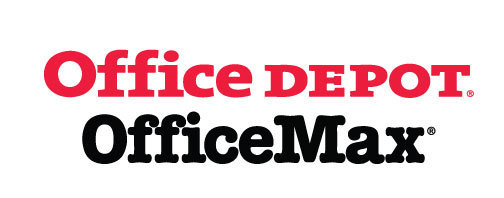Give Back to Northside

Purchase school and office supplies at Office Depot and OfficeMax, and Northside can receive 5% back in credits or FREE supplies. Simply provide our school ID 70031171 at checkout, online or in stores. There’s no cost to you!

 table div table+table+table+table+table+table+table+table+table+table+table+table+table+table+table+table+table+table+table+table+table+table+table+table+table+table+table+table+table+table+table+table+table+table+table+table+table+table+table+table+table+table+table+table+table+table+table+table+table+table+table+table+table+table+table+table+table+table+table+table+table+table+table+table+table+table+table+table+table+table+table+table+table+table+table+table+table+table+table+table+table+table+table+table+table+table+table+table+table div table{width:100%;padding:0}table div table+table+table+table+table+table+table+table+table+table+table+table+table+table+table+table+table+table+table+table+table+table+table+table+table+table+table+table+table+table+table+table+table+table+table+table+table+table+table+table+table+table+table+table+table+table+table+table+table+table+table+table+table+table+table+table+table+table+table+table+table+table+table+table+table+table+table+table+table+table+table+table+table+table+table+table+table+table+table+table+table+table+table+table+table+table+table+table+table div table img{width:96.23%;padding:0;float:none}table div table+table+table+table+table+table+table+table+table+table+table+table+table+table+table+table+table+table+table+table+table+table+table+table+table+table+table+table+table+table+table+table+table+table+table+table+table+table+table+table+table+table+table+table+table+table+table+table+table+table+table+table+table+table+table+table+table+table+table+table+table+table+table+table+table+table+table+table+table+table+table+table+table+table+table+table+table+table+table+table+table+table+table+table+table+table+table+table+table div table td{width:100%;padding:0 1.88% 18px}/* styles */Follow Us on Social Media

▪ NCP's Official Facebook Page
▪ Northside Prep Parents' Facebook Page, a closed group for parents, by parents, to share their knowledge of Northside
▪ NCP's Twitter Page
▪ Friends of Northside Facebook Page, for news everyone can view
 ▪ Northside Prep Parents' Facebook Page, a closed group for parents, by parents, to share their knowledge of Northside
 ▪ Friends of Northside Facebook Page, for news everyone can view
 table div table+table+table+table+table+table+table+table+table+table+table+table+table+table+table+table+table+table+table+table+table+table+table+table+table+table+table+table+table+table+table+table+table+table+table+table+table+table+table+table+table+table+table+table+table+table+table+table+table+table+table+table+table+table+table+table+table+table+table+table+table+table+table+table+table+table+table+table+table+table+table+table+table+table+table+table+table+table+table+table+table+table+table+table+table+table+table+table+table+table+table div table{width:100%;padding:0}table div table+table+table+table+table+table+table+table+table+table+table+table+table+table+table+table+table+table+table+table+table+table+table+table+table+table+table+table+table+table+table+table+table+table+table+table+table+table+table+table+table+table+table+table+table+table+table+table+table+table+table+table+table+table+table+table+table+table+table+table+table+table+table+table+table+table+table+table+table+table+table+table+table+table+table+table+table+table+table+table+table+table+table+table+table+table+table+table+table+table+table div table img{width:96.23%;padding:0;float:none}table div table+table+table+table+table+table+table+table+table+table+table+table+table+table+table+table+table+table+table+table+table+table+table+table+table+table+table+table+table+table+table+table+table+table+table+table+table+table+table+table+table+table+table+table+table+table+table+table+table+table+table+table+table+table+table+table+table+table+table+table+table+table+table+table+table+table+table+table+table+table+table+table+table+table+table+table+table+table+table+table+table+table+table+table+table+table+table+table+table+table+table div table td{width:100%;padding:0 1.88% 18px}/* styles */Seniors, click HERE for the Class of 2019 web page.

Northside’s website can be found HERE.

Friends of Northside's website can be found HERE.

To see all volunteer opportunities on one page, click HERE.

To ensure delivery of This Week at Northside, please add Thisweek@friendsofnorthside.org to your address book.

Please do not reply to this e-mail. Send your questions or comments to Friends of Northside.

Do you have news or team results to share with the Northside parent community?

 table div table+table+table+table+table+table+table+table+table+table+table+table+table+table+table+table+table+table+table+table+table+table+table+table+table+table+table+table+table+table+table+table+table+table+table+table+table+table+table+table+table+table+table+table+table+table+table+table+table+table+table+table+table+table+table+table+table+table+table+table+table+table+table+table+table+table+table+table+table+table+table+table+table+table+table+table+table+table+table+table+table+table+table+table+table+table+table+table+table+table+table+table+table div table{width:100%;padding:0}table div table+table+table+table+table+table+table+table+table+table+table+table+table+table+table+table+table+table+table+table+table+table+table+table+table+table+table+table+table+table+table+table+table+table+table+table+table+table+table+table+table+table+table+table+table+table+table+table+table+table+table+table+table+table+table+table+table+table+table+table+table+table+table+table+table+table+table+table+table+table+table+table+table+table+table+table+table+table+table+table+table+table+table+table+table+table+table+table+table+table+table+table+table div table img{width:96.23%;padding:0;float:none}table div table+table+table+table+table+table+table+table+table+table+table+table+table+table+table+table+table+table+table+table+table+table+table+table+table+table+table+table+table+table+table+table+table+table+table+table+table+table+table+table+table+table+table+table+table+table+table+table+table+table+table+table+table+table+table+table+table+table+table+table+table+table+table+table+table+table+table+table+table+table+table+table+table+table+table+table+table+table+table+table+table+table+table+table+table+table+table+table+table+table+table+table+table div table td{width:100%;padding:0 1.88% 18px}/* styles */